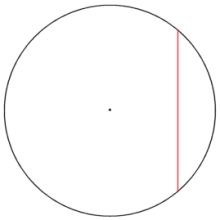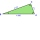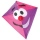# Circle chord

What is the length x of the chord circle of diameter 115 m, if the distance from the center circle is 11 m?

Result

x =  112.876 m

#### Solution:

$D=115 \ \text{m} \ \\ t=11 \ \text{m} \ \\ \ \\ r=D/2=115/2=\dfrac{ 115 }{ 2 }=57.5 \ \text{m} \ \\ \ \\ \ \\ (x/2)^2 +t^2=r^2 \ \\ \ \\ (x/2)^2=r^2 - t^2 \ \\ \ \\ x=2 \cdot \ \sqrt{ r^2-t^2 }=2 \cdot \ \sqrt{ 57.5^2-11^2 } \doteq \sqrt{ 12741 } \doteq 112.876 \doteq 112.876 \ \text{m}$Our examples were largely sent or created by pupils and students themselves. Therefore, we would be pleased if you could send us any errors you found, spelling mistakes, or rephasing the example. Thank you!

Leave us a comment of this math problem and its solution (i.e. if it is still somewhat unclear...):Be the first to comment!Tips to related online calculators
Pythagorean theorem is the base for the right triangle calculator.

#### You need to know the following knowledge to solve this word math problem:

We encourage you to watch this tutorial video on this math problem:

## Next similar math problems:

1. Concentric circles and chordIn a circle with a diameter d = 10 cm, a chord with a length of 6 cm is constructed. What radius have the concentric circle while touch this chord?
2. FlakesA circle was described on the square, and a semicircle above each side of the square was described. This created 4 "flakes". Which is bigger: the content of the central square or the content of four chips?
3. Height of the cuboidCuboid with a rectangular base, measuring 3 cm and 4 cm diagonal has a body 13 centimeters long. What is the height of the cuboid?
4. An equilateralAn equilateral triangle is inscribed in a square of side 1 unit long so that it has one common vertex with the square. What is the area of the inscribed triangle?
5. Spherical capThe spherical cap has a base radius of 8 cm and a height of 5 cm. Calculate the radius of a sphere of which this spherical cap is cut.
6. Medians in RTThe rectangular triangle ABC has a length of 10 cm and 24 cm. Points P, Q, R are the centers of the sides of this triangle. The perimeter of the PQR triangle is:
7. The right triangleThe right triangle ABC has a leg a = 36 cm and an area S = 540 cm2. Calculate the length of the leg b and the median t2 to side b.
8. Wall and body diagonalsThe block/cuboid has dimensions a = 4cm, b = 3cm and c = 12cm. Calculates the length of the wall and body diagonals.
9. The conicalThe conical candle has a base diameter of 20 cm and a side of 30 cm. How much dm ^ 3 of wax was needed to make it?
10. Cone roofHow many m2 of roofing is needed to cover a cone-shaped roof with a diameter of 10 m and a height of 4 m? Add an extra 4% to the overlays.Calculate the surface of a quadrilateral pyramid, which has a rectangular base with dimensions a = 8 cm, b = 6 cm and height H = 10 cm.Children have a kite on an 80m long rope, which floats above a place 25m from the place where children stand. How high is the dragon floating above the terrain?The regular triangular prism is 7 cm high. Its base is an equilateral triangle whose height is 3 cm. Calculate the surface and volume of this prism.Calculate the surface of a regular triangular prism, the edges of the base are 6 cm long and the height of the prism is 15 cm.The railway embankment 300 m long has a cross section of an isosceles trapezoid with bases of 14 m and 8 m. The trapezoidal arms are 5 m long. Calculate how much m3 of soil is in the embankment?Calculate the remaining sides of the right triangle if we know side b = 4 cm long and height to side c h = 2.4 cm.Kostka je vepsána do koule o poloměru r = 6 cm. Kolik procent tvoří objem kostky z objemu koule?